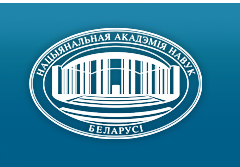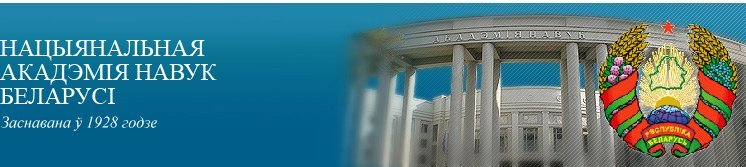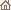Галоўная Навіны Аб акадэміі Дзейнасць акадэміі Члены акадэміі Арганізацыі Выданні акадэміі Мерапрыемствы Кантакты Электронныя звароты Даведачная інфармацыя Карта сайта

# Весці Нац. акадэміі навук Беларусі. Сер. фіз.-матэм. навук, 2003, No.3/ Выданні акадэміі / Навуковыя часопісы

Весці Нац. акадэміі навук Беларусі. Сер. фіз.-матэм. навук, 2003, No.3Весці Нацыянальнай акадэміі навук Беларусі СЕРЫЯ ФІЗІКА-МАТЭМАТЫЧНЫХ НАВУКВыдавец: Інстытут матэматыкі НАН Беларусі, Мінск, Рэспубліка Беларусь

### Нумар 3, 2003## ЗМЕСТ

### МАТЭМАТЫКА

Gorokhovik V. V.
On second-order optimality conditions for solutions of the optimization problem with inequality constraints. pp. 5--10

Summary: The paper presents a straightforward proof of the primal second order sufficient optimality condition for solutions of the optimization problem with inequality constraints. This proof does not use any dual variants of optimality conditions.

Demenchuk A. K.
On the existence of almost periodic solutions of linear differential systems in noncritical case. pp. 11--16

Summary: The existence problem for almost periodic partially irregular solutions of the system x = A(t)x + j(t), mod (A) Ç mod (k) = {0} in non-critical case is considered. The conditions for existence of such solutions are obtained.

Lyalikov A. S.
Approximation of continuous functions of bounded variation by rational operators of Vallee'-Poussin type. pp. 17--22

Summary: Continuous functions f having bounded variation on the given segment are considered. Rational operator of Vallee'-Poussin type is built, with poles being chosen depending on distribution of variation of f. Deviation of such operator from f is estimated.

Al'sevich L. A., Kastritsa O. A.
Explicit Calculation of Shift-Period-Mapping for Linear Systems with Block-reflective-matrix. pp. 23--25

Summary: The set of linear systems with block-reflective-function is constructed. For such systems a shift-period-mapping is given. The results apply to two-point boundary problem.

Makaruk S. F.
Boundary value problem on jump for a multiply connected domain with optimtimally situated internal domains. pp. 26--29

Summary: It is considered jump boundary value problem for multiply connected domains with unknown internal domains (inclusions). Distribution of the inclusions is determined by the optimality of the functional of effective conductivity and the constancy of their complete area. The solution is given in form of sums depending on centers of inclusions. For two and three inclusions optimal configurations are described, and the closed form solution is found in the important for application particular case.

Egorov A. D.
On the system of orthogonal polynomials on linear topological space with a non-Gaussian quasi-measure. pp. 30--33

Summary: The system of orthogonal polynomials on linear topological space with a non-Gaussian quasi-measure defined by arbitrary homogeneous (2p)-forms is constructed. Some special cases are considered.

Malyutin V. B.
Approximation for expectation from solution of nonlinear stochastic differential equation with anticommuting coefficients. pp. 34--37

Summary: The approximation for expectation from solution of nonlinear stochastic differential equation with anticom-muting coefficients is obtained.

Zherelo A. V.
On convergence of functional quadrature process for integrals on Poisson measure. pp. 38--42

Summary: The convergence conditions of functional quadrature process for continual integrals on Poisson measure in given class of functionals are obtained.

Bliznets I. M.
On the one of the class of the distributive lattices of t-closed w-saturated formations. pp. 43--45

Summary: It is established, that if the formation F is an element of height 3 of the lattice of all t-closed w-saturated formations, then its lattice of t-closed w-saturated subformations is distributive.

Semenchuk V. N., Mokeeva S. A.
Finite groups with F-subnomal and F-accessible subgroups. pp. 46--48

Summary: All groups considered are finite. In the paper we obtain a description of soluble hereditary saturated formations F such that the following condition holds: in any group G for any its two permutable F-subnormal subgroups H< and K the subgroup HK is also F-subnormal in G.

Sabuts A. V.
Criterions of existence of even integral positive definite quadratic forms. I. pp. 49--53

Summary: It is established that n-ary even integral positive definite quadratic forms with invariant factors 1,. . ., 1, d exist if only if the values of n and d satisfy one of the following conditions. First, d º +1(mod 4), d is not square, and n º 0(mod 4). Second, d = (2c - 1)2, c = 1,2 , . . . , and n º 0 (mod 8). Third, d º -1(mod 4), n º 2 (mod 4). Fourth, d = 0(mod 2), 2d is not square, and n º 1(mod 2); Fifth, d = 2c2, c = 1, 2, ..., and n º ±l(mod 8).

Kavalenok N. N.
Optimal control of dynamic systems with bang-bang functions. pp. 54--59

Summary: The optimal control problem with bang-bang principle is considered. Taking into account the specific structure of bang-bang controls the optimality condition is proved and an optimization algorithm is justified.

### ФІЗІКА

Bogush A. A.
On the quantum parameters and generators of the h-deformed group GLh(2.C). pp. 60--67

Summary: The description of the quantum (h-deformed) group GLh(2.C) in terms of the introduced quantum four-dimensional vector-parameters is given. The simple composition rules for these vector-parameters and, as a consequence, the related linearity relations are established and used. On this basis, it is shown that the realization of the transition GL(2.C) ® GLh(2.C) (gl(2.C) ® glh(2.C) ) may be reduced to the simple linear transformation of the group vector parameters (generators of the Lie algebra).

Ryabushko A. P., Nemanova I. T., Zhur T. A.
The relativistic translational motion of two rotating bodies comparable masses in the gravitational medium. pp. 68--73

Summary: In the post-Newtonian approximation the equations of the translational motion of two rotating bodies comparable masses in the gravitating medium have been integrated. Some novel relativistic effects due to the proper rotation of the bodies and the gravitational field of medium are detected.

Matsukovitsh S. M., Gavrilovich A. B.
The polarization characteristics of the radiation received from the diffusion spot source in the repeated dispersion conditions. pp. 74--78

Summary: Experimental research of the polarization characteristics of emanation in the disperse medium received from the spotted diffuse source have been carried out. The normal intensity angle distributions and polarization degrees with optic thickness that varies from 2.0 to 11.0 has been found. Positive and negative polarization changes with medium parameters variations have been analyzed.

Kamlyuk A. N., Nemtsov V. B.
Rotation of the plane of polarization for transverse waves propagating along the axis of biopolymers (like DNA). pp. 79--82

Summary: It is shown in the framework of the simple elastic rod with spiral structure model that the transversal wave propagation along the axis of biopolymers (like DNA) is followed by the rotation of the wave polarization plane.

Goncharenko E. A.
Coupled states of kinks for macroscopic chains. pp. 83--86

Summary: In the model with two real scalar fields of the fourth order potential an application of two and three soliton-like objects for description the macroscopic chains of atoms and clusters is considered.

Akimov A. I., Lebedev S. A., Letko A. K., Ivanova T. A.
The structure properties of the mixture Pr2-y--SryNixAl1-xO4. pp. 87--91

Summary: The effect of Ni/Al substitutions has been investigated for PrSrNixAl1-xO4 (0 £ x £ 0.7) compounds produced by conventional solid state reaction. Structural data for this system are reported. The unit cell parameter a increases when Al atoms are progressively replaced by Ni atoms but the parameter c decreases. A Rietvcld analysis of the room-temperature powder X-ray diffraction data showed that the tetrahedral distortion of the NiO6 increases with increasing x for 0 £ x £ 0.7 but the Pr(Sr) -- O(2) bond length decreases in the same interval.

Belevich N. N.
The obtaining of ZnSe films, their structural and optical properties. pp. 92--94

Summary: ZnSe films are obtained by method of thermal evaporation of the compound. Their structural and optical properties are explored at room temperature. It is shown that films have cubic structure. The width of a forbidden gape determined from optical absorption is equal 2.6 eV. On interference features of a transmission coefficient the refractive coefficient of films is explored, which is equal 2.90 ± 0.05 in the region of wavelengths from 500 to 1100 nm.

Mahnach V. I., Shved O. L.
Initial conditions of plasticity at the finite strains. pp. 95--99

Summary: It is shown that the plasticity function for the isotropic material represents the Cauchy task solution for an ordinary differential equation, which initial condition is defined by the experiment. For the particular case the solution has been obtained in the finite tensor-invariant form depending on the characteristics of the stress state. The plasticity curve is piecewise- regular, concave, the normal to this curve in the singular points are orthogonal.

### ІНФАРМАТЫКА

Tiunchik A. A.
Matching graph models of systolic-like algorithms on the basis of matrix representation of data structures. pp. 100--105

Summary: The formal approach for the construction of the spatial transformations for uniting decomposed graph models is proposed. This approach is based on the formalization of the description of input and output domains in graph models. The algorithms are split into the separate blocks and simpler graph models for the blocks is constructed. Matrix representation of the data structures that are to be transmitted between different blocks allows us to solve the problem of the spatial connection of different blocks in a systematic way. The existence of an appropriate spatial connection in a number of important cases is proven. Algebraic tools provide easy constructing complicated graph models.

Orlovich Yu. L.
Characterizations and recognition of some subclasses of well-covered modulo m graphs. pp. 106--114

Summary: The graph G is well-covered modulo m ³ 2 if |I1| º |I2| (mod m) for all maximal independent sets I1, I2 Í V(G). (The class of all such graphs is denoted by ZmW.) Characterizations of some hereditary subclasses of a class ZmW are obtained and the well-covered modulo m products of graphs are characterized. The polynomial solvability of a recognition problem G Î ZmW is proved for the following graphs: acyclic, claw-free, bounded maximum vertex degree.

Matskevich A. N.
Mathematical models casual non-stationary influences in the class of regular processes. pp. 115--117

Summary: The technique of synthesis and mathematical models of random non-stationary perturbations in the class of regular processes is represented.

### ВУЧОНЫЯ БЕЛАРУСI

Николай Александрович Борисевич (К 80-летию со дня рождения). С. 118--120
Націсніце
тут, каб прачытаць артыкул

Борис Борисович Бойко (К 80-летию со дня рождения). С. 121--123
Націсніце тут, каб прачытаць артыкул/ Выданні акадэміі / Навуковыя часопісы / Да пачатку старонкі

Распрацавана і падтрымліваецца Мікалаем М. Касцюковічам. Апошняе абнаўленне: 8 снежня 2006 г.
Створана пры ўдзеле Ігната І. Корсака
Капірайт © 2003-2006 Нацыянальная акадэмія навук Беларусі
Капірайт © 2003 Інстытут матэматыкі НАН Беларусі